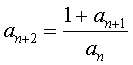The terms of a sequence are given by this equation where each term is found from the two preceding terms.

.For example if the first two terms are 2 and 5 then the next term is (1+5)/2=3 and the sequence is

2,  5,  3,  4/5,  3/5,  2,  5, … etc.

Investigate the sequences you get when you choose your own first two terms. What happens to your sequences?

Make a conjecture about the behaviour of sequences given by this rule.

1.juliusmukama says:
•toni says: